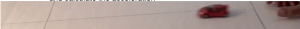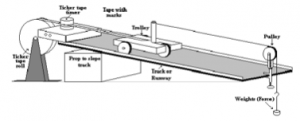### Newtons Second Law of Motion explained

Newton’s Second Law states that:
The acceleration of an object is in the same direction as the force applied and
is directly proportional to the size of the force.

We can see that the larger the force then the larger the acceleration. So the Sinclair C5 electric car does not accelerate as fast as the dragsters. In mathematical terms that is
Force is proportional to acceleration

or F is proportional to a

but we also know that if the mass is large then it is hard to accelerate,

so the rickshaw on the right may be rather hard to get going.

Mass is the constant of proportionality. The equation becomes:
F = m x a

To test the law we can accelerate a mass with a variety of constant forces on a very low friction surface

and then time it between two marks and calculate the accelerationExperiment on Newtons Second Law of Motionor use a ticker-timer to measure the changing velocity

The ramp in the experimental set up above is sloped just enough to compensate for the friction on the wheels of the trolley.

Download a pdf on all the notes for Newton's Laws here  Newton's Laws of Motion

Related pages are Newton's First Law

Newton's Third Law

Law of Momentum

Impulse and Momentum